# SQL Server Data Types

## SQL Server Data Types with Examples

In this article, I am going to discuss the SQL Server Data Types with examples. Please read our previous article before proceeding to this article where we discussed Creating, Altering and Deleting Database Tables in SQL Server with examples. As a developer, it is very important to understand SQL Server Data Types. As part of this article, we are going to discuss the following pointers in detail.

1. What is SQL Server Data Type?
2. Integer data types in SQL Server.
3. Decimal data types in SQL Server.
4. Understanding Money / Currency Data Type in SQL Server.
5. SQL Server Date and Time data types.
6. Character Data Type.
7. What are the differences between the fixed-length data type and a variable-length data type?
##### What is SQL Server Data Type?

The SQL Server Data Types are the attribute that specifies what types of data entered by the user such as integer, character, decimal, date time, etc. In SQL Server Database, each column of a table, all the local variables, and parameters must have a data type. The SQL Server supports the following data types

1. Integer data types
2. Decimal data types
3. Money / currency data types
4. Date and Time data types
5. Character data types
6. Binary data types
7. Special data types
##### Integer Data Types in SQL Server:

Integer Data Types are allowed only to hold integer types of values and this data type can be applied on EmpId, ProductCode, BracnchCode columns, etc. These data types are classified into 4 types based on their range and memory size as shown in the below image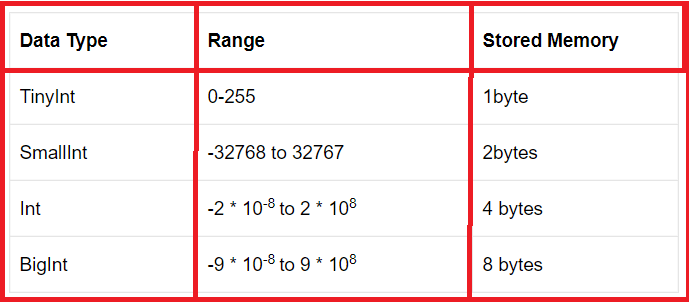##### Decimal Data Types in SQL Server:

These data types are allowed decimal point values only. The Decimal Data Type contains two types those are

1. Decimal (P, S)
2. Numeric (P, S)

But both are the same. Here P represents precision and S represent Scale and the default value of the Decimal data type is Decimal (18, 0) and also for Numeric (18, 0).

##### Precision (P):

The Precision is nothing but the maximum number of digits that we can store both to the left side and right side of the decimal point. Precision should have a value from 1 to 38. That is the minimum value is 1 and the maximum value is 38. The default value of precision is 18.

For example, if we have a decimal value such as 786.321 then the precision is 6 as we can see we have 3 digits in the left side of the decimal point as well as 3 digits at the right side of the decimal points.

Example: 285.21 here the precision is 5

##### Scale (S):

The scale is nothing but it just indicates the maximum number of decimal digits that we can store to the right of the decimal point. The scale must have a value from 0 through p (i.e. precision). We can specify the scale only if the precision is specified. The default scale is 0.

Example: 786.321 Here the scale is 3 as we have three digits that are present at the right side of the decimal point.

Example: 285.21 Scale is 2

The most important point that you need to remember is the default value of a scale is 0 and the maximum value of the scale depends on the precision value. The Decimal and Numeric data types are applied to product price, cost of the product, rate of the product, etc.

##### Let us see an example:

Price Decimal(6,2) Here the Precision is 6 so we can store a maximum of 6 digits. The scale is 2 so we can store a maximum of 2 digits at the right side of the decimal points. Please have a look at the below table where you will see which values are accepted and which are rejected.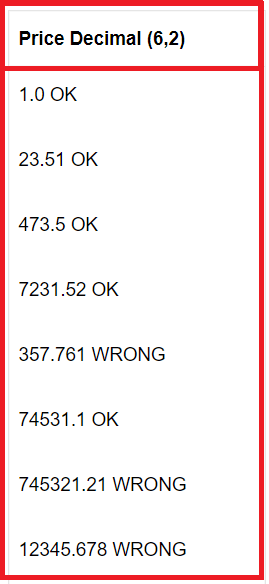Decimal Data Type Size in SQL Server: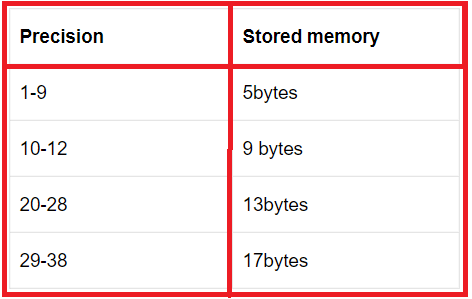Note: numeric is functionally equivalent to decimal.

##### SQL Server Money / Currency Data Type:

These data types are used to accept currency format values into a table column.

The money data type again classified into two types.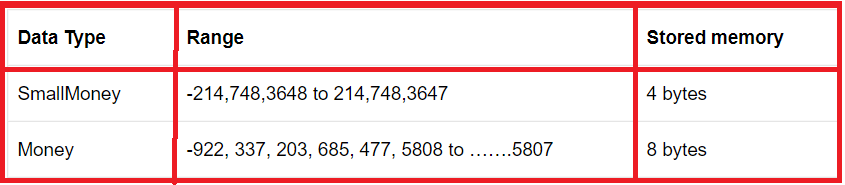These data types are applying to employee salary, student fee columns, etc.

##### SQL Server Date and Time data types:

Date and Time data types are used to store a particular date and time information. These are applying on the date of joining, date of birth, hire date, order date columns, etc. Date and time data types again classified into 3 types, such as

1. Date: This data type will accept date format information only. The default format of the date data type is ‘YYYY/MM/DD
2. Time: It allows time format information only. The default format of the time data type is ‘hh:mm:ss.ms
3. DateTime: It allows date and time format information. The default format of DateTime data type is ‘YYYY/MM/DD hh:mm: ss.ms’.
##### Character Data Types in SQL Server:

Character data types are allowed characters and integer format values. These data types can be applied to employee name, student name, and product name columns, etc. Character data types again classified into two types, those are Unicode data types and Non-Unicode data types.

1. Non Unicode data types: char (Size), varchar (size/max), Text
2. Unicode data types: nchar(size), nvarchar(size), ntext
##### Char (size):
1. It is a fixed-length data type (static data type).
2. It will store the data type in the Non-Unicode mechanism that means it will occupy 1byte for 1 character.
3. The maximum length of the char data type is from 1 to 8000 bytes.

Disadvantages: memory wastage because size cannot be changed at runtime.

##### Varchar (size/max):
1. It is a variable-length data type (dynamic data type) and will store the character in a non-Unicode manner that means it will take 1 byte for 1 character.
2. The maximum length of the varchar data type is from 1 to 8000 bytes
##### Text:
1. The text data type is the old version data type of SQL Server and similar to the varchar(max) data type.

Note: The above 3 data types come under the Non-Unicode mechanism and supported to localized data (English culture data only).

##### What are the differences between the fixed-length data type and a variable-length data type?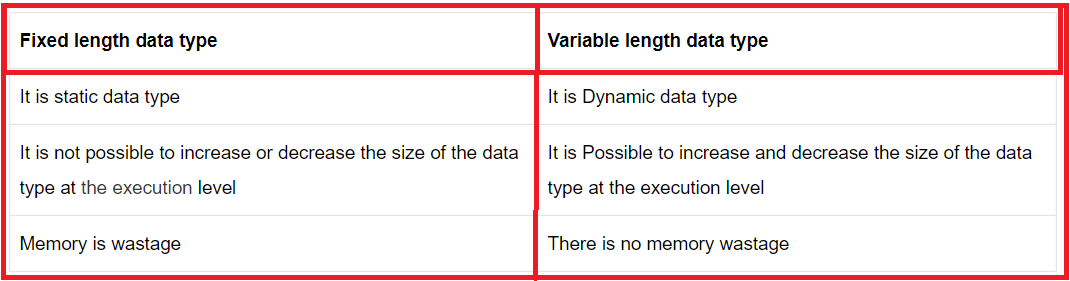nchar(Size) data type:

1. It is a fixed-length data type and will stores the characters in the Unicode manner that means it will take 2bytes memory per a single character.
2. The maximum length of nchar data type is from up to 4000bytes.
###### Nvarchar(size/max) data type:
1. It is a variable-length data type and will store the data type in the Unicode manner that means it will occupy 2bytes of memory per a single character.
2. The maximum length of nvarchar data type is from up to 4000 bytes.
###### ntext data type:
1. It is an old version data type of SQL Server and similar to nvarchar(max/size) data type.
2. Here ‘n’ represents the national.
###### Binary data type:

These data types are used to store image files, audio files, and video files into a database location.

Binary data types again classified into three types, such as

###### Binary(size):
1. It is a fixed-length data type and will store binary format information (0,1).
2. The maximum length of the binary data type is from up to 8000 bytes.
###### Varbinary(size/max):
1. It is a variable-length data type and will store the information in the form of binary format.
2. The maximum length of the varbinary data is from up to 8000bytes (we can store 2gb information).
###### Image data type:
1. It is an old version data type of SQL Server and similar to the varbinary data type.

Note: Instead of text, ntext and image data type we use varchar(max), nvarchar(max) and varbinary(max) data types in latest versions SQL Server.

###### Boolean Type:
1. To hold the Boolean values it provides a bit data type that can take a value of 1, 0, or NULL.

Note: The string values TRUE and FALSE can be converted to bit values. TRUE is converted to 1 and FALSE is converted to 0.

###### Special data types:

SQL Server supports the following special data types:

###### Sql_varient data type:

This data type will support all data types data except text, ntext and image data type because these are the old version data type of SQL Server.

For example:

 X Sql_varient 101 Pkr 32.68 13579 22/12/2112 101000110
###### XML data type:

It is used for storing XML file information (Tag-based programming information).

###### SQL Server Cursor Data Type:

The cursor is an object data type that will allocate a reference memory in the server-side for storing temporary table information.

###### Timestamp Datatype in SQL Server:

The Timestamp is a data type in SQL Server that exposes automatically generated, unique binary numbers within a database. The size of the Timestamp data type is 8 bytes. In SQL Server, we generally use this timestamp on the column of a row to determine whether any data in the row has been changed since the last time it was read. If we do any changes to the data of the row, then the value of the timestamp column of that row is updated. If the data of the row is unchanged then the timestamp value is the same as when it was previously read.

###### Unique identifier:

The Unique Identifier is a 16-byte GUID. We can initialize this by using the newid() built-in SQL Server function. The most important point that you need to be remembered is that the GUID values are uniquely identified in a database. That means it is going to be unique within the database.

In the next article, I am going to discuss Constraint in SQL Server with examples. Here, in this article, I try to explain SQL Server Data Types with examples. I hope this article will help you with your needs.